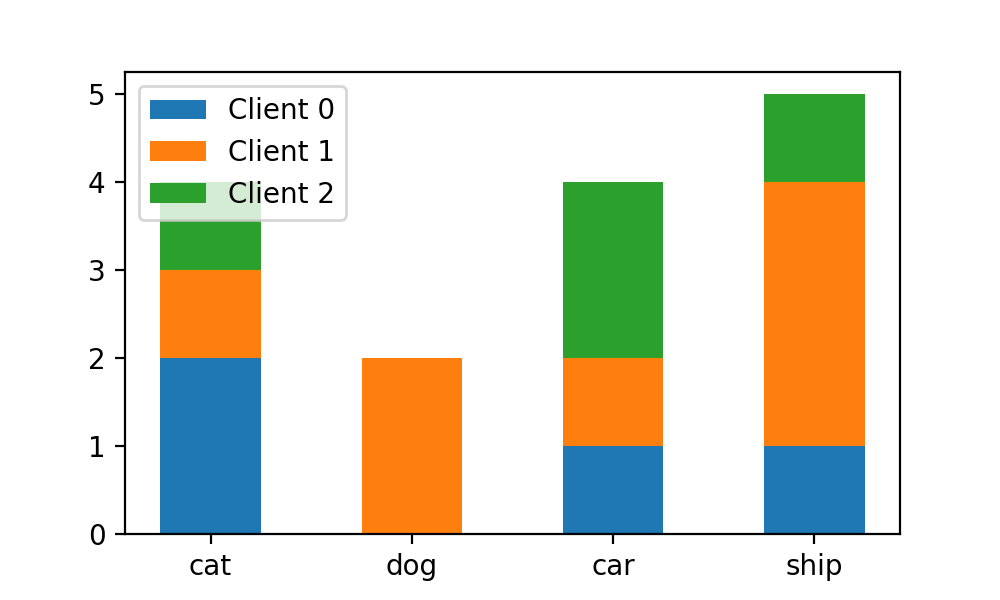# Matplotlib直方图绘制中的参数bins和rwidth

2022-02-03 19:10 2491 查看

## 情境引入

`matplotlib.pyplot`
`hist`

`cat`
,
`dog`
,
`car`
,
`ship`
。这个数据集已经被不均衡地划分到4个任务节点(client)上，如像下面表示：

```N_CLIENTS = 3
num_cls, classes = 4, ['cat', 'dog', 'car', 'ship']
train_labels = [0, 3, 2, 0, 3, 2, 1, 0, 3, 3, 1, 0, 3, 2, 2] #数据集的标签列表

client_idcs = [slice(0, 4), slice(4, 11), slice(11, 15)]
# 数据集样本在client上的划分情况```

```import matplotlib.pyplot as plt
import numpy as np

plt.figure(figsize=(5,3))
plt.hist([train_labels[idc]for idc in client_idcs], stacked=False,
bins=num_cls,
label=["Client {}".format(i) for i in range(N_CLIENTS)])

plt.xticks(np.arange(num_cls), classes)
plt.legend()
plt.show()```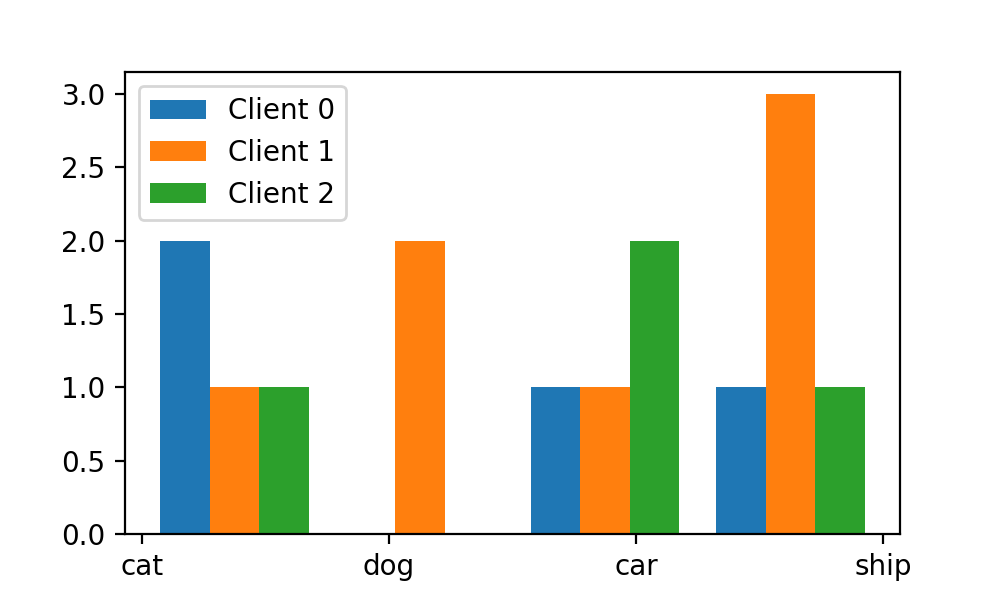`bins`

## bins 参数

`hist`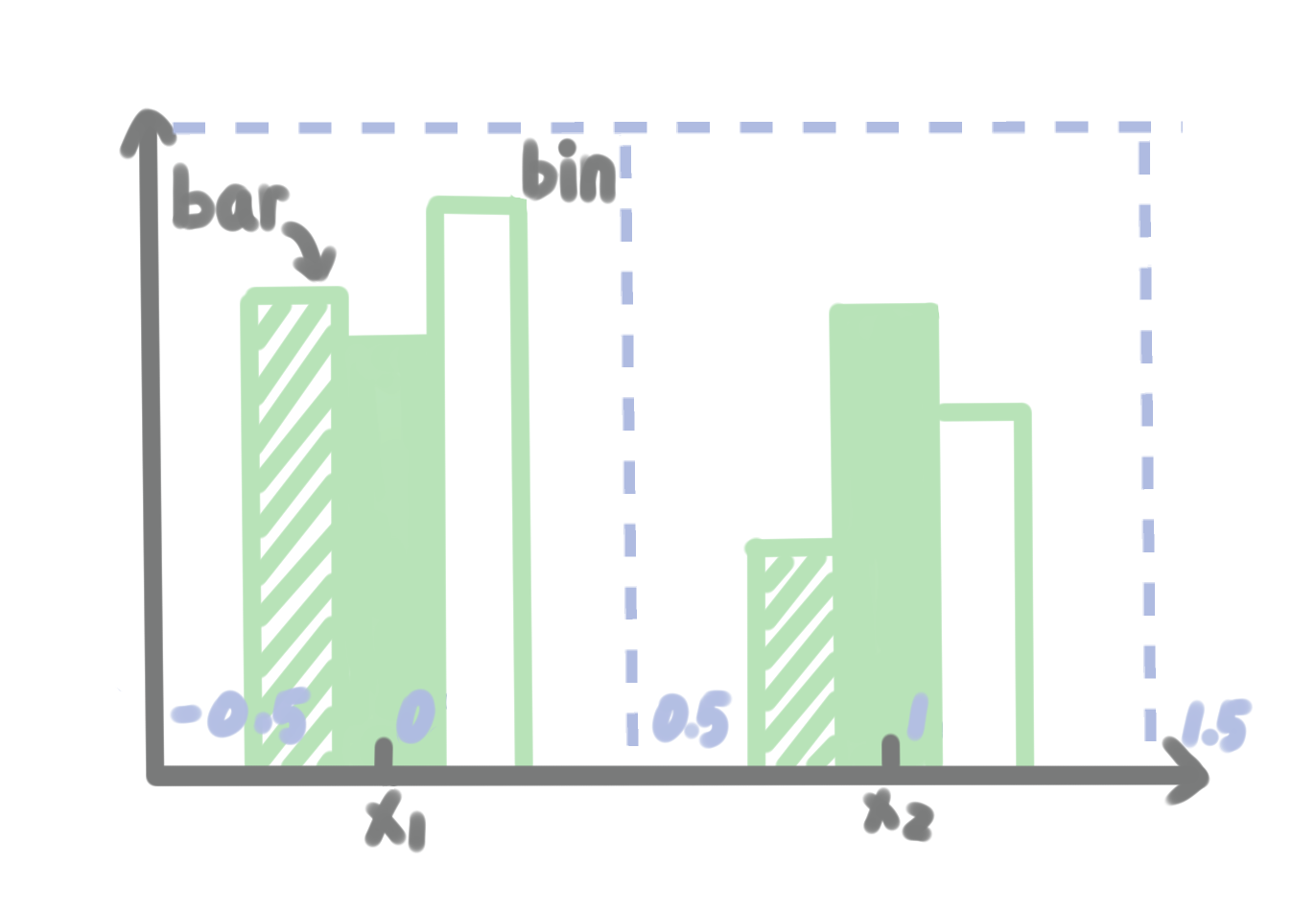`hist`

`hist`

`matplotlib`

`bins`

bins: int or sequence or str, default: rcParams["hist.bins"] (default: 10)

If bins is an integer, it defines the number of equal-width bins in the range.

If bins is a sequence, it defines the bin edges, including the left edge of the first bin and the right edge of the last bin; in this case, bins may be unequally spaced. All but the last (righthand-most) bin is half-open. In other words, if bins is:

`[1, 2, 3, 4]`

then the first bin is [1, 2) (including 1, but excluding 2) and the second [2, 3). The last bin, however, is [3, 4], which includes 4.

If bins is a string, it is one of the binning strategies supported by numpy.histogram_bin_edges: 'auto', 'fd', 'doane', 'scott', 'stone', 'rice', 'sturges', or 'sqrt'.

`bins`

`bins`

`bins`

`hist`

`[0, 1, 2, 3]`
。如果我们将序列设置为
`[0, 1, 2, 3, 4]`

`[1, 2)`
，第2个绘图区域对应的位置是
`[1, 2)`
,第三个绘图区域对应的位置是
`[2, 3)`
，依次类推。

`[-0.5, 0.5)`
，第二个区域的区间为
`[0.5, 1.5)`
，依次类推。则最终的
`bins`

`[-0.5, 0.5, 1.5, 2.5, 3.5]`
。于是，我们将
`hist`

```plt.hist([train_labels[idc]for idc in client_idcs], stacked=False,
bins=np.arange(-0.5, 4, 1),
label=["Client {}".format(i) for i in range(N_CLIENTS)])```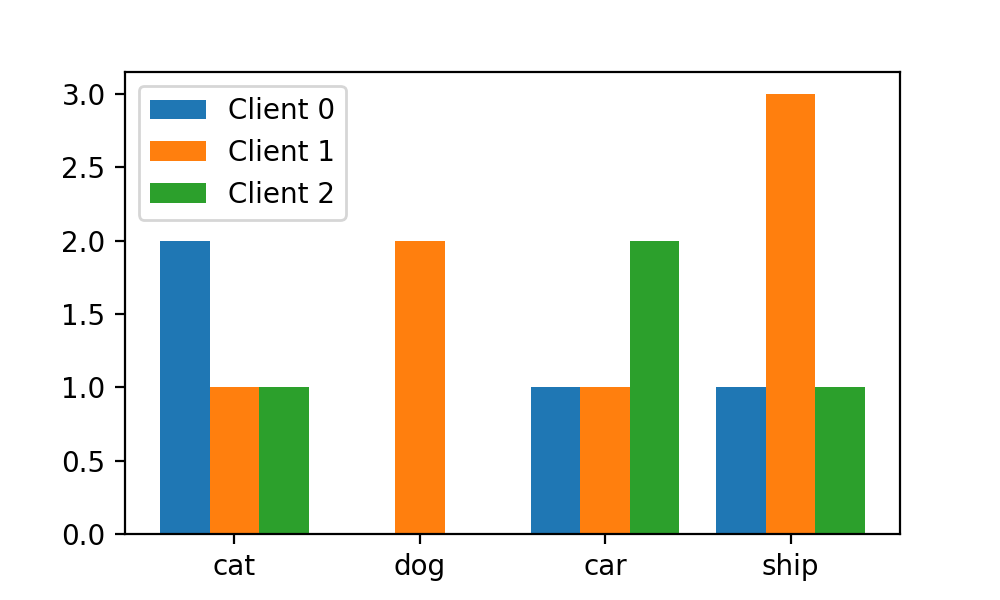## stacked参数

`stacked`

`stacked`

`True`
:

```plt.hist([train_labels[idc]for idc in client_idcs],stacked=True
bins=np.arange(-0.5, 4, 1),
label=["Client {}".format(i) for i in range(N_CLIENTS)])```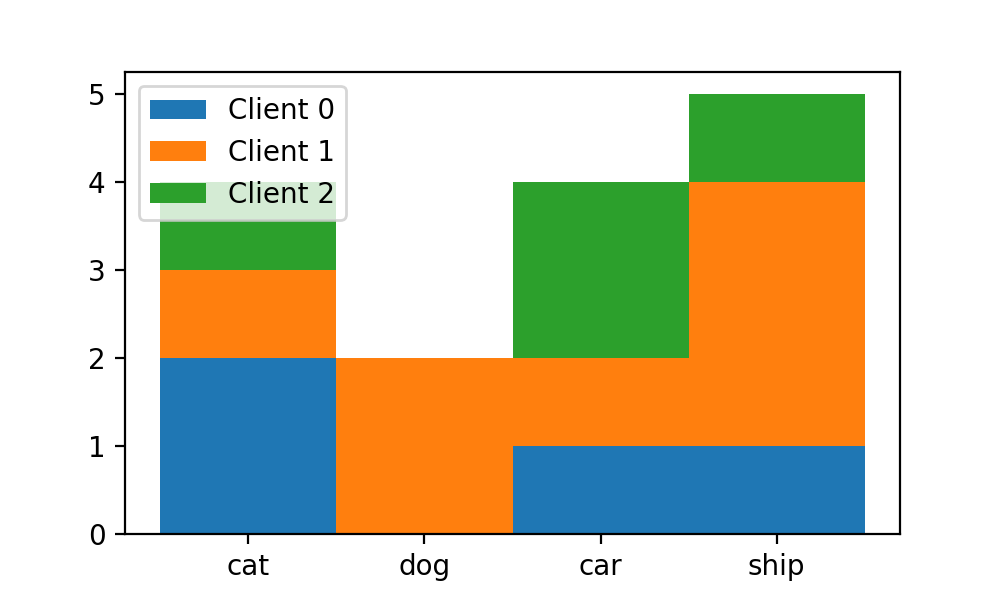`bins`

`bins`

`r_width`

## rwidth 参数

`rwidth`

rwidth float or None, default: None

The relative width of the bars as a fraction of the bin width. If None, automatically compute the width.

Ignored if histtype is 'step' or 'stepfilled'.

`rwidth`

```plt.hist([train_labels[idc]for idc in client_idcs],stacked=True,
bins=np.arange(-0.5, 4, 1), rwidth=0.5,
label=["Client {}".format(i) for i in range(N_CLIENTS)])```